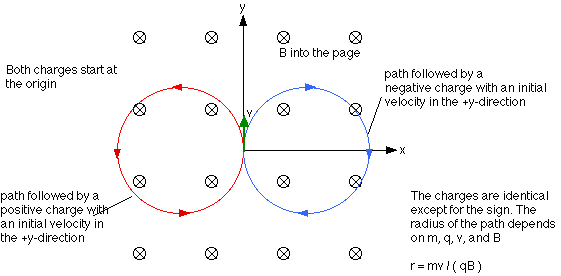Magnetic fields and how to make them

7-14-99

Magnetism

There is a strong connection between electricity and magnetism. With electricity, there are positive and negative charges. With magnetism, there are north and south poles. Similar to charges, like magnetic poles repel each other, while unlike poles attract.

An important difference between electricity and magnetism is that in electricity it is possible to have individual positive and negative charges. In magnetism, north and south poles are always found in pairs. Single magnetic poles, known as magnetic monopoles, have been proposed theoretically, but a magnetic monopole has never been observed.

In the same way that electric charges create electric fields around them, north and south poles will set up magnetic fields around them. Again, there is a difference. While electric field lines begin on positive charges and end on negative charges, magnetic field lines are closed loops, extending from the south pole to the north pole and back again (or, equivalently, from the north pole to the south pole and back again). With a typical bar magnet, for example, the field goes from the north pole to the south pole outside the magnet, and back from south to north inside the magnet.

Electric fields come from charges. So do magnetic fields, but from moving charges, or currents, which are simply a whole bunch of moving charges. In a permanent magnet, the magnetic field comes from the motion of the electrons inside the material, or, more precisely, from something called the electron spin. The electron spin is a bit like the Earth spinning on its axis.

The magnetic field is a vector, the same way the electric field is. The electric field at a particular point is in the direction of the force a positive charge would experience if it were placed at that point. The magnetic field at a point is in the direction of the force a north pole of a magnet would experience if it were placed there. In other words, the north pole of a compass points in the direction of the magnetic field.

One implication of this is that the magnetic south pole of the Earth is located near to the geographic north pole. This hasn't always been the case: every once in a while (a long while) something changes inside the Earth's core, and the earth's field flips direction. Even at the present time, while the Earth's magnetic field is relatively stable, the location of the magnetic poles is slowly shifting.

The symbol for magnetic field is the letter B. The unit is the tesla (T).

The magnetic field produced by currents in wires

The simplest current we can come up with is a current flowing in a straight line, such as along a long straight wire. The magnetic field from a such current-carrying wire actually wraps around the wire in circular loops, decreasing in magnitude with increasing distance from the wire. To find the direction of the field, you can use your right hand. If you curl your fingers, and point your thumb in the direction of the current, your fingers will point in the direction of the field. The magnitude of the field at a distance r from a wire carrying a current I is given by:Currents running through wires of different shapes produce different magnetic fields. Consider a circular loop with a current traveling in a counter-clockwise direction around it (as viewed from the top). By pointing your thumb in the direction of the current, you should be able to tell that the magnetic field comes up through the loop, and then wraps around on the outside, going back down. The field at the center of a circular loop of radius r carrying a current I is given by: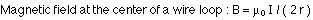For N loops put together to form a flat coil, the field is just multiplied by N: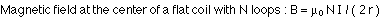If a number of current-carrying loops are stacked on top of each other to form a cylinder, or, equivalently, a single wire is wound into a tight spiral, the result is known as a solenoid. The field along the axis of the solenoid has a magnitude of: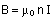where n = N/L is the number of turns per unit length (or, in other words, the total number of turns over the total length).

The force on a charged particle in a magnetic field

An electric field E exerts a force on a charge q. A magnetic field B will also exert a force on a charge q, but only if the charge is moving (and not moving in a direction parallel to the field). The direction of the force exerted by a magnetic field on a moving charge is perpendicular to the field, and perpendicular to the velocity (i.e., perpendicular to the direction the charge is moving).

The equation that gives the force on a charge moving at a velocity v in a magnetic field B is: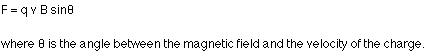This is a vector equation : F is a vector, v is a vector, and B is a vector. The only thing that is not a vector is q.

Note that when v and B are parallel (or at 180°) to each other, the force is zero. The maximum force, F = qvB, occurs when v and B are perpendicular to each other.

The direction of the force, which is perpendicular to both v and B, can be found using your right hand, applying something known as the right-hand rule. One way to do the right-hand rule is to do this: point all four fingers on your right hand in the direction of v. Then curl your fingers so the tips point in the direction of B. If you hold out your thumb as if you're hitch-hiking, your thumb will point in the direction of the force.

At least, your thumb points in the direction of the force as long as the charge is positive. A negative charge introduces a negative sign, which flips the direction of the force. So, for a negative charge your right hand lies to you, and the force on the negative charge will be opposite to the direction indicated by your right hand.

In a uniform field, a charge initially moving parallel to the field would experience no force, so it would keep traveling in straight-line motion, parallel to the field. Consider, however, a charged particle that is initially moving perpendicular to the field. This particle would experience a force perpendicular to its velocity. A force perpendicular to the velocity can only change the direction of the particle, and it can't affect the speed. In this case, the force will send the particle into uniform circular motion. The particle will travel in a circular path, with the plane of the circle being perpendicular to the direction of the field.

In this case, the force applied by the magnetic field ( F = qvB ) is the only force acting on the charged particle. Using Newton's second law gives:The particle is undergoing uniform circular motion, so the acceleration is the centripetal acceleration:

a = v2 / r

so, q v B = m v2 / r

A factor of v cancels out on both sides, leaving

q B = m v / r The radius of the circular path is then: r = m v / (q B)

A particle that is initially moving at some angle between parallel and perpendicular to the field would follow a motion which is a combination of circular motion and straight-line motion...it would follow a spiral path. The axis of the spiral would be parallel to the field.

To understand this, simply split the velocity of the particle into two components: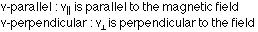The field does not affect v-parallel in any way; this is where the straight line motion comes from. On the other hand, the field and v-perpendicular combine to produce circular motion. Superimpose the two motions and you get a spiral path.

Working in three dimensions

With the force, velocity, and field all perpendicular to each other, we have to work in three dimensions. It can be hard to draw in 3-D on a 2-D surface such as a piece of paper or a chalk board, so to represent something pointing in the third dimension, perpendicular to the page or board, we usually draw the direction as either a circle with a dot in the middle or a circle with an X in the middle.

Think of an arrow with a tip at one end and feathers at the other. If you look at an arrow coming toward you, you see the tip; if you look at an arrow going away from you, you see the X of the feathers. A circle with a dot, then, represents something coming out of the page or board at you; a circle with an X represents something going into the page or board.

The following diagram shows the path followed by two charges, one positive and one negative, in a magnetic field that points into the page: